# WebGL Essentials: Part II

This post is part of a series called WebGL Essentials.
WebGL Essentials: Part I
WebGL Essentials: Part III

This article will build on to the framework introduced in part one of this mini-series, adding a model importer and a custom class for 3D objects. You will also be introduced to animation and controls. There's a lot to go through, so let's get started!

This article relies heavily on the first article, so, if you haven't read it yet, you should start there first.

The way WebGL manipulates items in the 3D world is by using math formulas known as transformations. So, before we start building the 3D class, I will show you some of the different kinds of transformations and how they are implemented.

## Transformations

There are three basic transformations when working with 3D objects.

• Moving
• Scaling
• Rotating

Each of these functions can be performed on either the X, Y, or Z axis, making a total possibility of nine basic transformations. All of these affect the 3D object's 4x4 transformation matrix in different ways. In order to perform multiple transformations on the same object without overlapping problems, we have to multiply the transformation into the object's matrix and not apply it to the object's matrix directly. Moving is the easiest to do, so let's start there.

### Moving A.K.A. "Translation"

Moving a 3D object is one of the easiest transformations you can do, because there is a special place in the 4x4 matrix for it. There's no need for any math; just put the X, Y and Z coordinates in the matrix and your done. If you are looking at the 4x4 matrix, then it's the first three numbers in the bottom row. Additionally, you should know that positive Z is behind the camera. Therefore, a Z value of -100 places the object 100 units inwards on the screen. We will compensate for this in our code.

In order to perform multiple transformations, you can't simply change the object's real matrix; you must apply the transformation to a new blank matrix, known as an identity matrix, and multiply it with the main matrix.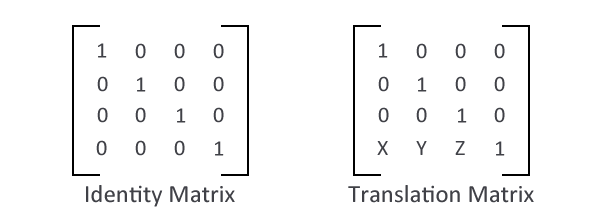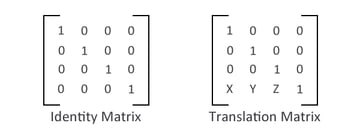Matrix multiplication can be a bit tricky to understand, but the basic idea is that each vertical column is multiplied by the second matrix's horizontal row. For example, the first number would be the first row multiplied by the other matrix's first column. The second number in the new matrix would be the first row multiplied by the other matrix's second column, and so on.

The following snippet is code I wrote for multiplying two matrices in JavaScript. Add this to your .js file that you made in the first part of this series:

I don't think this requires any explanation, as it's just the necessary math for matrix multiplication. Let's move on to scaling.

### Scaling

Scaling a model is also fairly simple - it's simple multiplication. You have to multiply the first three diagonal numbers by whatever the scale is. Once again, the order is X, Y, and Z. So, if you want to scale your object to be two times bigger in all three axes, you would multiply the first, sixth, and eleventh elements in your array by 2.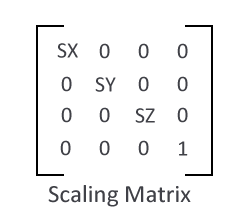### Rotating

Rotating is the trickiest transformation because there is a different equation for each of the three axis. The following image shows the rotation equations for each axis: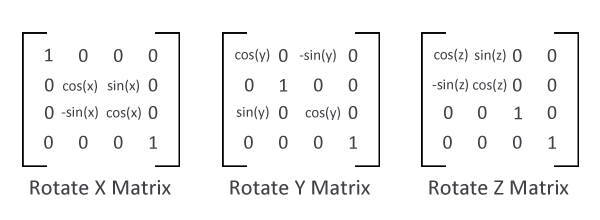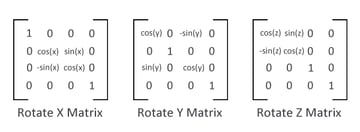Don't worry if this picture doesn't make sense to you; we'll review the JavaScript implementation soon.

It's important to note that it matters what order you perform the transformations; different orders produce different results.

It's important to note that it matters what order you perform the transformations; different orders produce different results. If you first move your object and then rotate it, WebGL will swing your object around like a bat, as opposed to rotating the object in place. If you rotate first and then move your object, you will have an object in the specified location, but it will face the direction that you entered. This is because the transformations are performed around the origin point - 0,0,0 - in the 3D world. There is no right or wrong order. It all depends on the effect you are looking for.

It could require more than one of each transformations to make some advanced animations. For instance if you want a door to swing open on its hinges, you would move the door so that its hinges are on the Y axis (ie 0 on both the X and Z axis). You would then rotate on the Y axis so the door will swing on its hinges. Finally, you would move it again to the desired location in your scene.

These type of animations are a bit more custom-made for each situation, so I'm not going to make a function for it. I will, however, make a function with the most basic order which is: scaling, rotating, and then moving. This insures everything is in the specified location and facing the right way.

Now that you have a basic understanding of the math behind all of this and how animations work, let's create a JavaScript data type to hold our 3D objects.

## GL Objects

Remember from the first part of this series that you need three arrays in order to draw a basic 3D object: the vertices array, the triangles array, and the textures array. That will be the base of our data type. We also need variables for the three transformations on each of the three axes. Finally, we need a variables for the texture image and to indicate whether the model has finished loading.

Here is my implementation of a 3D object in JavaScript:

I've added two separate "ready" variables: one for when the image is ready, and one for the model. When the image is ready, I will prepare the model by converting the image into a WebGL texture and buffer the three arrays into WebGL buffers. This will speed up our application, as apposed to buffering the data in every draw cycle. Since we will convert the arrays into buffers, we need to save the number of triangles in a separate variable.

Now, let's add the function that will calculate the object's transformation matrix. This function will take all the local variables and multiply them in the order that I mentioned earlier (scale, rotation, and then translation). You can play around with this order for different effects. Replace the //Add Transformation function Here comment with the following code:

Because the rotation formulas overlap one another, they have to be performed one at a time. This function replaces the MakeTransform function from the last tutorial, so you can remove it from your script.

## OBJ Importer

Now that we have our 3D class built, we need a way to load the data. We'll make a simple model importer that will convert .obj files into the necessary data to make one of our newly created GLObject objects. I am using the .obj model format because it stores all the data in a raw form, and it has very good documentation on how it stores the information. If your 3D modeling program doesn't support exporting to .obj, then you can always create an importer for some other data format. .obj is a standard 3D file type; so, it shouldn't be a problem. Alternatively you can also download Blender, a free cross-platform 3D modeling applications that does support exporting to .obj

In .obj files, the first two letters of every line tell us what kind of data that line contains. "v" is for a "vertex coordinates" line, "vt" is for a "texture coordinates" line, and "f" is for the mapping line. With this information, I wrote the following function:

This function accepts the name of a model and a callback function. The callback accepts four arrays: the vertex, triangle, texture, and normal arrays. I haven't yet covered normals, so you can just ignore them for now. I will go through them in the follow-up article, when we discuss lighting.

The importer starts by creating an XMLHttpRequest object and defining its onreadystatechange event handler. Inside the handler, we split the file into its lines and define a few variables. .obj files first define all the unique coordinates and then defines their order. That is why there are two variables for the vertices, textures, and normals. The counter variable is used to fill in the triangles array because .obj files define the triangles in order.

Next, we have to go through each line of the file and check what kind of line it is:

The first three line types are fairly simple; they contain a list of unique coordinates for the vertices, textures and normals. All we need to do is push these coordinates into their respective arrays. The last kind of line is a bit more complicated because it can contain multiple things. It could contain just vertices, or vertices and textures, or vertices, textures, and normals. As such, we have to check for each of these three cases. The following code does this:

This code is more long than it is complicated. Although I covered the scenario where the .obj file only contains vertex data, our framework requires vertices and texture coordinates. If a .obj file contains only vertex data, you will have to manually add the texture coordinate data to it.

Let's now pass the arrays to the callback function and finish up the LoadModel function:

Something you should watch out for is that our WebGL framework is fairly basic and only draws models that are made out of triangles. You may have to edit your 3D models accordingly. Luckily, most 3D applications have a function or plug-in to triangulate your models for you. I made a simple model of a house with my basic modeling skills, and I will include it in the source files for you to use, if you are so inclined.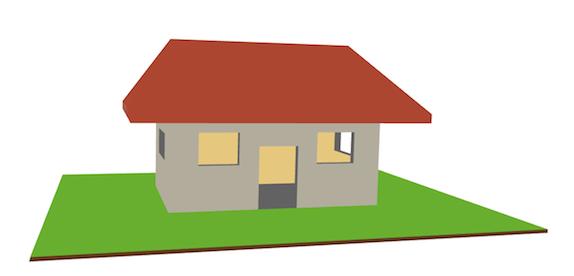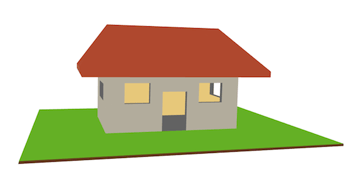Now let's modify the Draw function from the last tutorial to incorporate our new 3D object data type:

The new draw function first checks if the model has been prepared for WebGL. If the texture has loaded, it will prepare the model for drawing. We will get to the PrepareModel function in a minute. If the model is ready, it will connect its buffers to the shaders and load the perspective and transformation matrices like it did before. The only real difference is that it now takes all the data from the model object.

The PrepareModel function just converts the texture and data arrays into WebGL compatible variables. Here is the function; add it right before the draw function:

Now our framework is ready and we can move on to the HTML page.

## The HTML Page

You can erase everything that is inside the script tags as we can now write the code more concisely thanks to our new GLObject data type.

This is the complete JavaScript:

We load a model and tell the page to update it at around thirty times per second. The Update function rotates the model on the Y axis, which is accomplished by updating the object's Y Rotation property. My model was a bit too big for the WebGL scene and it was backwards, so I needed to perform some adjustments in code.

Unless you are making some kind of cinematic WebGL presentation, you are probably going to want to add some controls. Let's look at how we can add some keyboard controls to our application.

## Keyboard Controls

This is not really a WebGL technique as much as a native JavaScript feature, but it is handy for controlling and positioning your 3D models. All you have to do is add an event listener to the keyboard's keydown or keyup events and check which key was pressed. Each key has a special code, and a good way to find out which code corresponds to the key is to log the key codes to the console when the event fires. So go to the area where I loaded the model, and add the following code right after the setInterval line:

This will set the function handleKeyDown to handle the keydown event. Here is the code for the handleKeyDown function:

All this function does is update the object's properties; the WebGL framework takes care of the rest.

## Conclusion

We're not done! In the third and final part of this mini-series, we will review different kinds of lighting, and how to tie it all up with some 2D stuff!